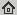# Fourier Analysis in Polar and Spherical Coordinates

Technical Report 1, IIF-LMB, Computer Science Department, University of Freiburg (1), 2008
Abstract: In this paper, polar and spherical Fourier Analysis are defined as the decomposition of a function in terms of eigenfunctions of the Laplacian with the eigenfunctions being separable in the corresponding coordinates. Each eigenfunction represents a basic pattern with the wavenumber in- dicating the scale. The proposed transforms provide an effective radial decomposition in addition to the well-known angular decomposition. The derivation of the basis functions is compactly presented with an emphasis on the analogy to the normal Fourier transform. The relation between the polar or spherical Fourier transform and normal Fourier transform is explored. Possible applications of the proposed transforms are discussed.

## BibTex reference

```@TechReport{WRB08,
author       = "Q.Wang and O.Ronneberger and H.Burkhardt",
title        = "Fourier Analysis in Polar and Spherical Coordinates",
institution  = "IIF-LMB, Computer Science Department, University of Freiburg ",
number       = "1",
year         = "2008",
url          = "http://lmb.informatik.uni-freiburg.de/Publications/2008/WRB08"
}
```